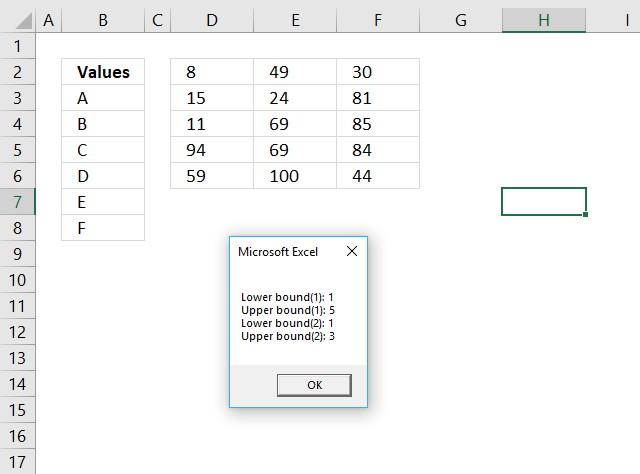Author: Oscar Cronquist Article last updated on October 22, 2018The Lbound and Ubound functions calculate the size of of an array. The Lbound returns the lower limit of an array and the Ubound function returns the upper limit.

```Sub Macro4()

Rng = Range("D2:F6").Value

MsgBox "Lower bound(1): " & LBound(Rng, 1) & vbNewLine & _
"Upper bound(1): " & UBound(Rng, 1) & vbNewLine & _
"Lower bound(2): " & LBound(Rng, 2) & vbNewLine & _
"Upper bound(2): " & UBound(Rng, 2)

End Sub
```

The macro above populates variable Rng with values from range D2:F6, the Lbound and Ubound functions then return the lower and upper limits for the first dimension (rows) and the second dimension (columns).

### Excel Function VBA Syntax

LBound( arrayname,  [dimension] )

UBound( arrayname,  [dimension] )

### Arguments

 arrayname Required. The array variable. [dimension] Optional. Which dimension to use, the default value is 1.

The following macro creates array variable  TestArray with one dimension, lower limit of 1 and upper limit of 3.

```Sub Macro1()

Dim TestArray(1 To 3)
MsgBox LBound(TestArray) & vbNewLine & UBound(TestArray)

End Sub
```

This macro creates array variable TestArray with two dimensions, the first dimension has the lower limit of 1 and an upper limit of 3, the second dimension has the lower limit of 1 and the upper limit of 10.

```Sub Macro2()

Dim TestArray(1 To 3, 1 To 10)
MsgBox "Lower bound(1): " & LBound(TestArray, 1) & vbNewLine & _
"Upper bound(1): " & UBound(TestArray, 1) & vbNewLine & _
"Lower bound(2): " & LBound(TestArray, 2) & vbNewLine & _
"Upper bound(2): " & UBound(TestArray, 2)

End Sub
```

This macro populates array variable TestArray with values from cell range B3:B8, the first dimension has the lower limit of 1 and an upper limit of 6, the second dimension has the lower limit of 1 and the upper limit of 1.

```Sub Macro3()

Rng = Range("B3:B8").Value
MsgBox "Lower bound(1): " & LBound(Rng, 1) & vbNewLine & _
"Upper bound(1): " & UBound(Rng, 1) & vbNewLine & _
"Lower bound(2): " & LBound(Rng, 2) & vbNewLine & _
"Upper bound(2): " & UBound(Rng, 2)

End Sub
```